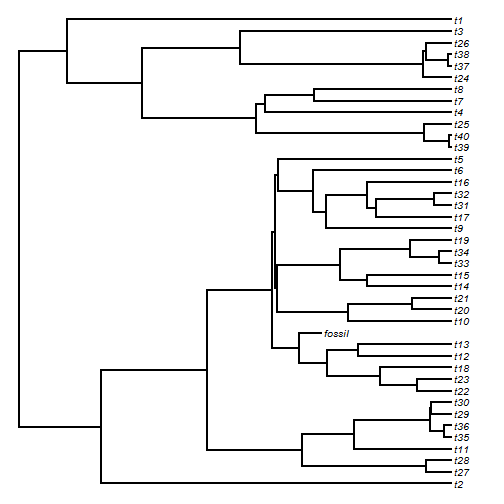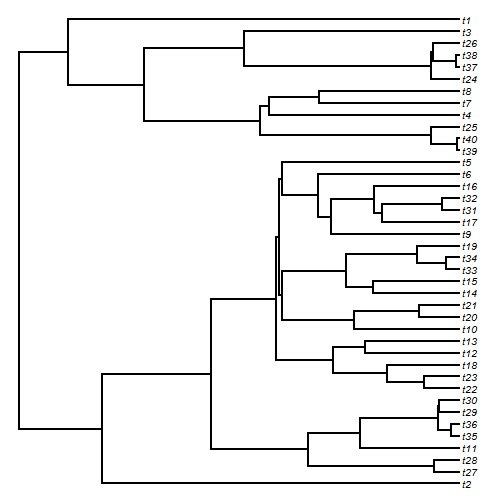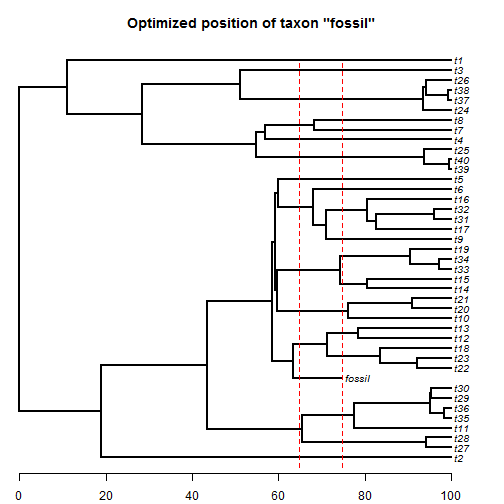## Sunday, December 7, 2014

### Fossil version of locate.yeti to place taxa in a tree using continuous characters

I have now posted development code for a fossil version of the phytools function `locate.yeti`, called `locate.fossil`.

This function uses ML to place a single fossil tip into a tree based on continuous character data. It allows the user to impose a `time.constraint`, in the form of a time range for the fossil. If the age of the fossil is known precisely, this can just be a single numeric value; and if the user wants to supply it (rather than as a height above the root) as a time before present, this should be given as a negative scalar. Note that `time.constraint` constrains only the height of the tip, and not at all the height of the attachment of the terminal edge to the tree - except inasmuch as this must precede in time the height of the tip of course! The user can also supply an `edge.constraint` which constrains the edges of the tree to which the tip can attach. Obviously, these must be compatible with `time.constraint` or the function will not work.

Here's a demo of estimation using simulated data:

``````library(phytools)
library(mnormt)
## here is our true tree (normally unknown)
plotTree(true.tree,ftype="i",fsize=0.8)
````````````## simulate data
X<-fastBM(true.tree,nsim=20)
## drop tip to recreate the empirical backbone tree
tree<-drop.tip(true.tree,"fossil")
plotTree(tree,ftype="i",fsize=0.8)
````````````## now let's make an age constraint based on the real (known) age of
## the fossil lineage:
h<-nodeHeights(true.tree)[true.tree\$edge[,2]==which(true.tree\$tip.label=="fossil"),2]
max(nodeHeights(tree))-h
``````
``````##  30.14439
``````
``````age.range<-c(h-5,h+5)
age.range
``````
``````##  64.85561 74.85561
``````
``````## load source code for locate.fossil
source("locate.fossil.R")
mltree<-locate.fossil(tree,X,time.constraint=age.range,plot=FALSE)
``````
``````## Optimizing the phylogenetic position of fossil using ML. Please wait....
## Done.
``````
``````## plot the result
plotTree(mltree,mar=c(2.1,0.1,3.1,0.1),ftype="i",fsize=0.8)
axis(1)
obj<-lapply(age.range,function(x,tree) lines(rep(x,2),
c(0,Ntip(tree)+1),col="red",lty="dashed"),tree=tree)
title(paste("Optimized position of taxon \"",setdiff(rownames(X),tree\$tip.label),
"\"",sep=""))
``````The function also contains an option to plot the progress of the likelihood search. This is somewhat difficult to illustrate on the web. (I attempted to use `saveGIF` from the 'animation' package, but was having trouble for some unknown reason.)

That's it for now.

1.A few queries:

1) What's the minimum size of the branch the fossil taxon attaches to the tree with? Do you allow for zero-length branches? (A ZLB would essentially mean its a direct ancestor of an extant lineage...)

2) Would it be possible to constrain the search to only consider scenarios where the taxon is connected by a ZLB? Then one could compare the likelihood scores (using an LRT, I think) to test if a fossil specimen was likely to be a direct ancestor or not (which is a feasible hypothesis and an pertinent question in some cases...).

2.1) Yes, zero.

2) Not presently, but theoretically straightforward.

This is a prototype function right now - it computes the likelihood correctly, but I don't know about optimization and/or constraints on the optimization. I will be working on it more.

3.Ah, well, cool function nonetheless!

Note: due to the very large amount of spam, all comments are now automatically submitted for moderation.